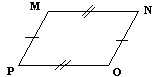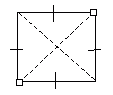Email us to get an instant 20% discount on highly effective K-12 Math & English kwizNET Programs!

#### Online Quiz (WorksheetABCD)

Questions Per Quiz = 2 4 6 8 10

 Polygon with fours sides are called quadrilaterals. A quadrilateral with two pairs of parallel and equal sides is called a parallelogram. A parallelogram with 4 right angles and 4 sides equal is a square. A parallelogram with 4 right angles and 2 pairs of opposite equal sides is a rectangle. A quadrilateral with only 1 pair of parallel sides is called trapezoid. A parallelogram with four equal sides is called a rhombus. The diagonals of a parallelogram are not equal. Directions: Answer the following. Also draw all the kinds of quadrilaterals and write their properties on a sheet of paper.
 Q 1: Quadrilaterals are polygons with _____(The prefixthree sidesfour sidestwo sides Q 2: In this quadrilateral: trapezoid which lines are parallelXY and WZYW and WZXW and WZ Q 3: A quadrilateral with only 1 pair of parallel sides is calledtrapezoidparallelogramsquare Q 4: The diagonals of parallelogramare of equal lengthare not of equal length Q 5: The diagonals of rectangleare not of equal lengthare of equal length Q 6: The opposite sides of a parallelogram areintersect at pointperpendicularparallel Q 7: A quadrilateral with 2 pairs of parallel sides is calledsquaretrapezoidparallelogram Q 8: What is a parallelogram with 4 right angles and 4 sides equaltrapezoidrectanglesquare Question 9: This question is available to subscribers only! Question 10: This question is available to subscribers only!